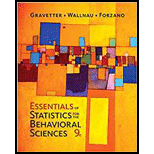Chapter 8, Problem 7PEssentials of Statistics for The B...

9th Edition
Frederick J Gravetter + 2 others
ISBN: 9781337098120

Solutions

Chapter
SectionEssentials of Statistics for The B...

9th Edition
Frederick J Gravetter + 2 others
ISBN: 9781337098120
Textbook Problem

Babcock and Marks (2010) review survey data from 2003-2005, and obtained an average of μ – 14 hours per week spent studying by full-time students at four-year colleges in the United States. To determine whether this average has changed in recent years, a researcher selected a sample of n – 64 of today’s college students and obtained an average of M = 12.5 hours. If the standard deviation for the distribution is π - 4.8 hours per week, does this sample indicate a significant change in the number of hours spent study­ing? Use a two-tailed test with α = .05.

To determine
Whether the sample indicates a significant change in the number of hours spent studying or not.

Explanation

Given info:

Samples mean (M) is given as 12.5, population mean is given as 14, standard deviation is given as 4.8, alpha level is given as 0.05 and sample size is given as 64.

Calculation:

The null hypothesis states that there is no effect of the treatment and alternative hypothesis states opposite of null hypothesis. As given in the question that population mean is 14 and two tailed test is used, so the null hypothesis and alternative hypothesis for this question are:

H0:μ=14H1:μ14

The alpha level is given in the question as 0.05 and need to find boundaries of the critical region. As given in the question that two tailed hypothesis test used, so acceptance region is:

p=1α2=10.052=10.025=0.975

The z-score critical value at 0.975 or at α=0.05 is ±1.96 using the z table

Still sussing out bartleby?

Check out a sample textbook solution.

See a sample solution

The Solution to Your Study Problems

Bartleby provides explanations to thousands of textbook problems written by our experts, many with advanced degrees!

Get Started

Find the limit. limx1ex3x

Calculus: Early Transcendentals

Find the derivative of the function. y=cossin(tanx)

Single Variable Calculus: Early Transcendentals, Volume I

Study Guide for Stewart's Multivariable Calculus, 8th

An integral for the solid obtained by rotating the region at the right about the x-axis is:

Study Guide for Stewart's Single Variable Calculus: Early Transcendentals, 8th